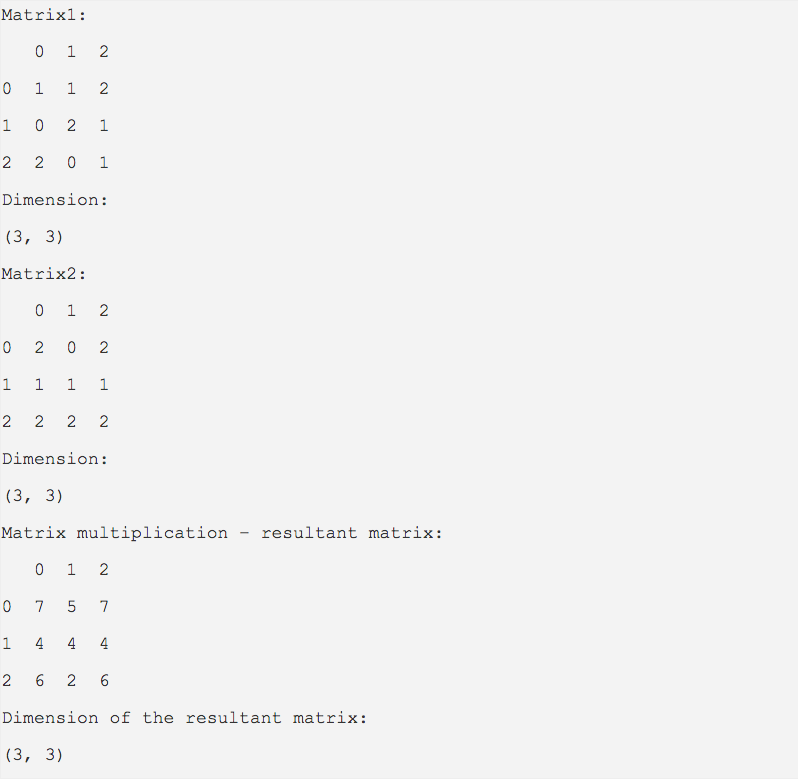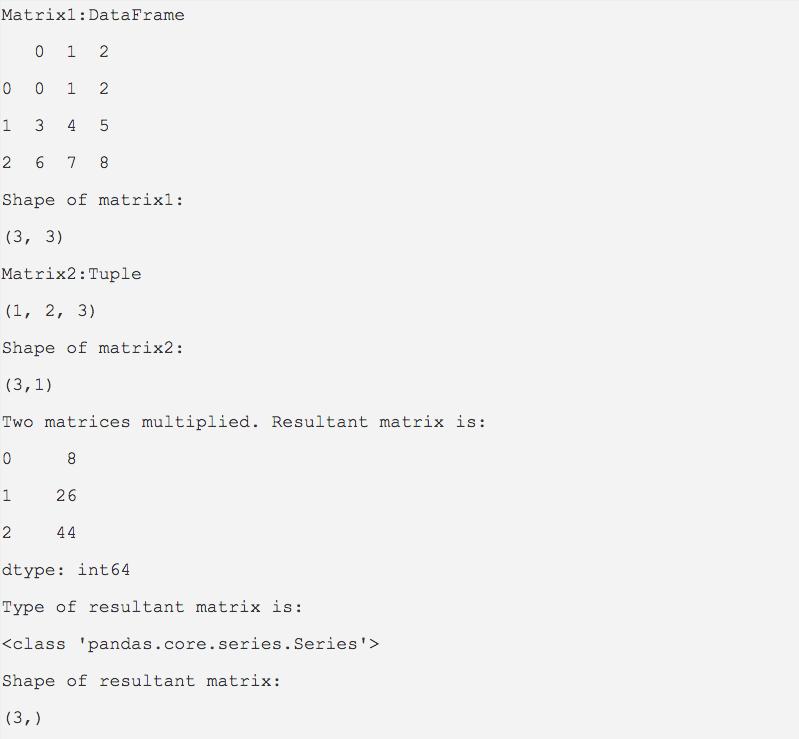# Matrix Multiplication Using Pandas DataFrames

## Overview:

• The dot() method of pandas DataFrame class does a matrix multiplication between a DataFrame and another DataFrame, a pandas Series or a Python sequence and returns the resultant matrix.
• When two matrices one with columns 'i' and rows 'j' and another with columns 'j' and rows 'k' are multiplied - 'j' elements of the rows of matrix one are multiplied with the 'j' elements of the columns of the matrix two and added to create a value in the resultant matrix with dimension (ixk).

## Example - Multiplying two matrices of same dimensions:

 import pandas as pd   # Data matrix1 = [(1, 1, 2),            (0, 2, 1),            (2, 0, 1)];   matrix2 = [(2, 0, 2),            (1, 1, 1),            (2, 2, 2)];   # Data loaded into pandas DataFrames dataFrame1 = pd.DataFrame(data=matrix1); dataFrame2 = pd.DataFrame(data=matrix2);   print("Matrix1:"); print(dataFrame1); print("Dimension:"); print(dataFrame1.shape);   print("Matrix2:"); print(dataFrame2); print("Dimension:"); print(dataFrame2.shape);   # Multiply the matrices: Matrix1 and Matrix2 result = dataFrame1.dot(dataFrame2);   # Print the result of matrix multiplication print("Matrix multiplication – resultant matrix:"); print(result); print("Dimension of the resultant matrix:"); print(result.shape);

## Output:## Example:  Multiplying matrices of different dimensions

 import pandas as pd   data1 = [(0,1,2),         (3,4,5),         (6,7,8)];   matrix_3x1 = (1, 2, 3); matrix_3x3 = pd.DataFrame(data=data1);   # Note that in this example dot is invoked with a plain python tuple. # Not with a pandas DataFrame...not with a pandas series resultant  = matrix_3x3.dot(matrix_3x1);   print("Matrix1:DataFrame") print(matrix_3x3); print("Shape of matrix1:") print(matrix_3x3.shape);   print("Matrix2:Tuple") print(matrix_3x1); print("Shape of matrix2:") print("(%d,%d)"%(len(matrix_3x1), 1));   print("Two matrices multiplied. Resultant matrix is:") print(resultant);   print("Type of resultant matrix is:") print(type(resultant));   print("Shape of resultant matrix:") print(resultant.shape);

## Output: